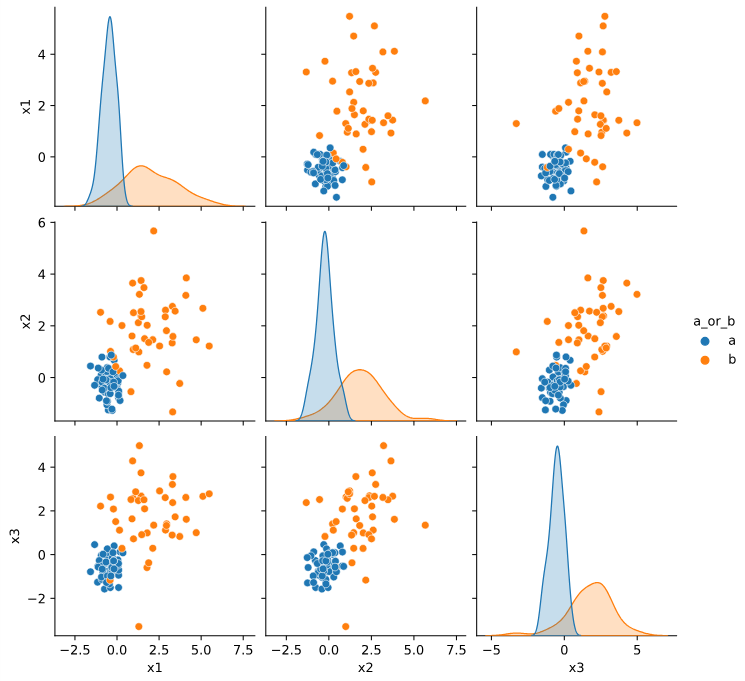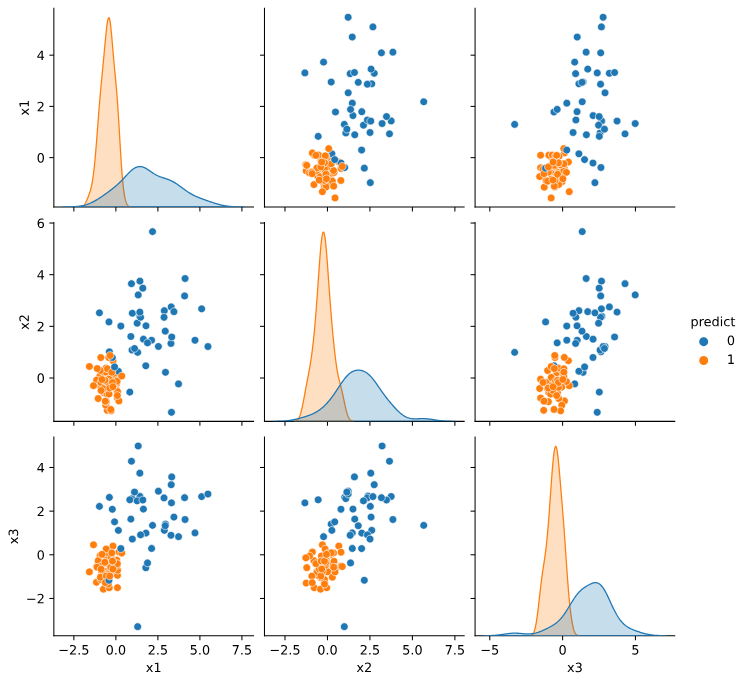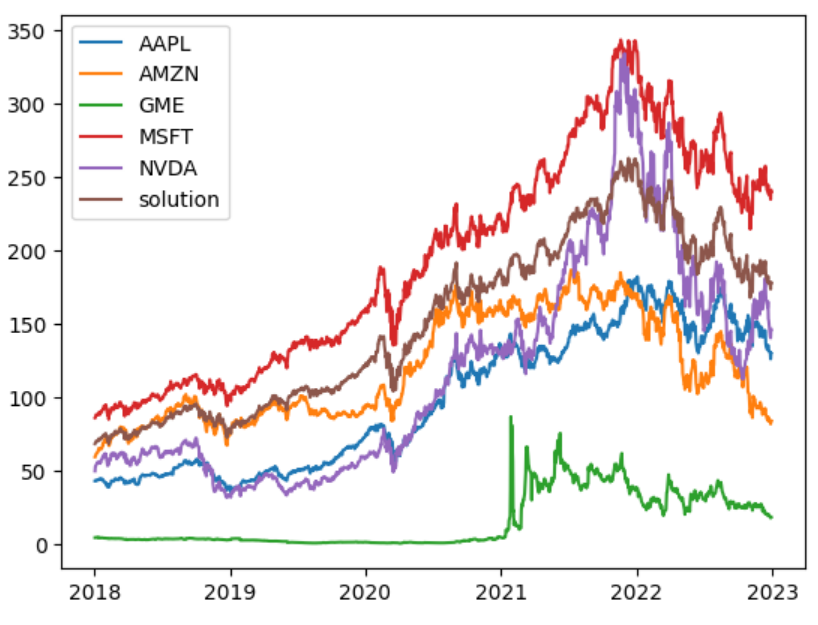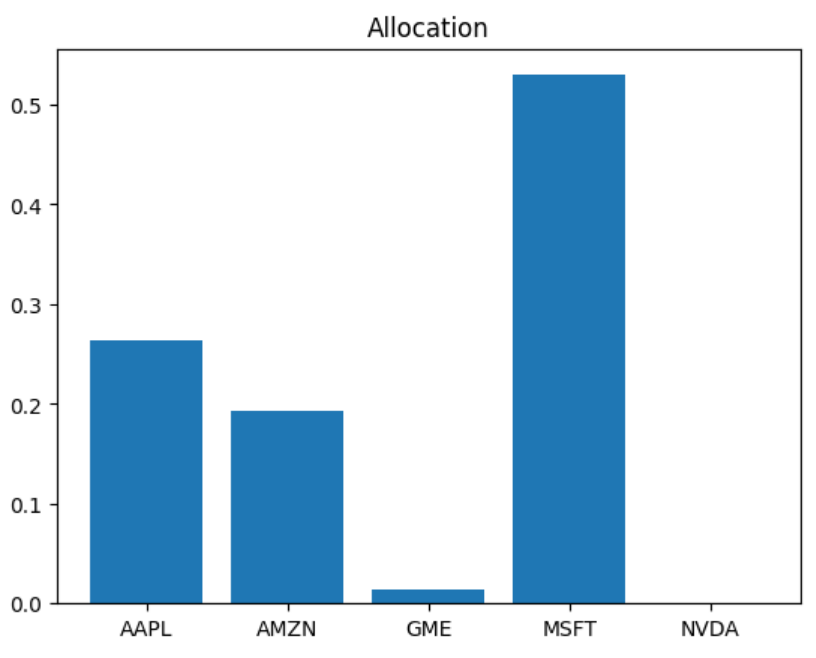# Best Frenemies: Julia and Python

| Sep 5, 2023 min read

Julia and Python are often seen as competitors, but in this video I want to show the integration between them. Using the PythonCall and the JuliaCall packages we can call Julia from Python and Python from Julia. Check the video out or check edited transcript below.

Don’t forget to like and subscribe.There are currently two ways to integrate Python in Julia and Julia in Python. These two main packages are PyCall and PythonCall and their corresponding Python package. I have talked about PyCall in a series of blog posts in the past, comparing Python being improved by C++ and Python being improved by Julia up to the point that we actually made the Julia code faster than the C++ code in this specific instance. Now I’m going to talk about the PythonCall package and the corresponding JuliaCall package which are newer.

## Calling Python from Julia

So very briefly, you install PythonCall, And it installs a Python version for you. Use the conda package to install the packages that you need for Python call. So here I’m installing PythonCall in this Pluto environment. I’m using Pkg and I’m saying conda add seaborne and conda add scikit learn.

``````using PythonCall, Random, DataFrames, CondaPkg
using Pkg
``````

So I’m just installing these two packages. And here I am calling some code. This is Julia code as normal. Essentially I’m creating a hundred by three matrix with some distribution that depends on whether the Data is from the type A or the type B, which is essentially fake data for a classification problem.

``````Random.seed!(0)
n = 100
p = 3
a_or_b = rand([:a, :b], n)
μ = Dict(:a => -0.5, :b => 1.5)
σ = Dict(:a => 0.5, :b => 1.5)
X = [
randn() * σ[a_or_b[i]] + μ[a_or_b[i]]
for i = 1:n, j = 1:p
]
df = DataFrame(
[X a_or_b],
[["x\$i" for i = 1:p]; "a_or_b"],
)
sns = pyimport("seaborn")
sns.pairplot(pytable(df), hue="a_or_b")
``````

So if you look at these and you have seen the classification problem before, you probably recognize the seaborne package that plots all of the three axis. and the classes of these points. So the way that we do that, so all the way up to here, this is all just Julia, nothing new. But then we just use pyimport seaborn to import that Python package. And then we call sns.pairplot. And now you’re just calling some Python code.

I’m just calling the pairplot function from Seaborn. I’m giving it a data frame, but converted using pytable, which is part of the package that we just installed. So this is how you can easily just use a Python package inside Julia.

Here is the output:Down here I have a bit longer example now using the scikit-learn package. So I’m using sklearn.naive_bayes. I then have the gaussianNB constructor. I create a classifier. And down here I fit this classifier, the input of this classifier is a Julia matrix and a Julia array of integers. So it just works. And then when I call predict, I have a new vector, y hat.

``````nb = pyimport("sklearn.naive_bayes")
clf = nb.GaussianNB()

X = Matrix(df[:, 1:end-1])
y = Int.(df[:, end] .== :a)

clf.fit(X, y)
ŷ = clf.predict(X)
df_predict = copy(df)
df_predict[!, :predict] = pyconvert(Vector, ŷ)
sns.pairplot(pytable(df_predict), hue="predict")
``````

The way that I chose to plot this is again, giving it to pyplot. I just convert this vector. This is a Python vector. So I convert it to a Julia vector using the pyconvert function.

Here is the output:## Calling Julia from Python

So to use Julia from inside Python, you can just install the Julia call package and just `pip install juliacall`. You will have access to import main, and you can give it a name like `jl`, and you can see here that it installs a Julia for you, so you don’t have to worry about that.

``````from juliacall import Main as jl
``````

To install Julia packages, you want to use The juliapkg package. So it’s also `pip install juliapkg`. And then you have to say here, juliapkg.Add, and give the package name and the UUID. These might be harder to get but the easiest way will be to just open Julia and say, add JuMP and ask for the status and it’ll list this information for you.

``````import juliapkg
juliapkg.resolve()
``````

And when you add your packages, you say resolve to get them installed. And you can also use a json file and list this information on the json file, which I ignore here. So we just have a single notebook. So this install this jump and ipopt packages and jump and ipopt, you know, are packages for optimization.

So to just show this package working, I’m gonna implement a simple optimization model to find the best investment if I try to base myself on historic information of these five stocks. So this is, is known as the Markowitz model, which is a simple model. So don’t use it in your stock selection.

But anyway, so just so you know, I’m using yfinance. So this is all Python. I download closing data from these stocks. I I’m just plotting for you to see and computing the returns. I have to use the mean value and the covariance matrix. Just so I can compute the risk and the expected return of this selection.

``````import yfinance as yf

data = data["Close"]

returns = data.diff() / data
returns = returns[1:]

mean_returns = returns.mean()
std_returns = returns.cov()
``````

And now we start using Julia. So `jl.seval`, which is a string evaluation using jump, using Ipopt. Then you can say things like model equals Model(Ipopt.Optimizer). You could use another syntax here if you try to get the jump value. So `jl.JuMP`, and you can just call model directly but then you have to write more.

So this was just simpler, not going to say it’s faster. I don’t know one way to pass data around. If you want to pass the actual data to Julia, you say JL dot the variable name equals data. And then it, this data that is just a Python thing is now also a Julia thing. I’m actually not using this for anything, but just so you know.

The Markowitz model needs the mean return, the expected return, and this covariance matrix. And what it does is it has one variable for each one of my stock, and I want to minimize the risk. So if you don’t know this, that’s fine. This is Julia, that’s the important thing. And I want an expected return here that I’m just saying it needs to be positive. These are constraints and here you are investing all of your money. And that’s it. That’s the whole model. You optimize this model. All of this is Julia. `jl.seval`. I’m obtaining the value of the allocation and here I’m getting the objective value, which is how much risk my selection is on, which doesn’t matter a lot for us. The important part is that I can plot these things now back into Python. So that’s it. This is like just a Julia insertion in my Python notebook.

``````jl.seval("using JuMP")
jl.seval("using Ipopt")
model = jl.seval("model = Model(Ipopt.Optimizer)")

jl.data = data

jl.n = data.shape
jl.mean_r = mean_returns.values
jl.std_r = std_returns.values
jl.seval("@variable(model, x[1:n] >= 0)")
jl.seval("@objective(model, Min, dot(x, std_r, x))")
jl.seval("@constraint(model, dot(x, mean_r) / n >= 0)")
jl.seval("@constraint(model, sum(x) == 1)")

jl.seval("optimize!(model)")

x = jl.seval("value.(x)")
f = jl.seval("objective_value(model)")

model
``````

Now I’m back to Python. So here I can analyze the solution. So this brown curve. So we had a pretty good result. Here you can see the allocation. It’s mostly Microsoft stocks and hindsight is 20/20, so please don’t use this for your investment. This is not investment suggestion or advising of any kind.

``````import numpy as np

sol_data = data.copy()
sol_data['solution'] = np.matmul(data.values, x)
plt.plot(sol_data)
plt.legend(sol_data.columns)
````````````plt.bar(range(0, len(x)), x)
plt.xticks(range(0, len(x)), data.columns)
plt.title("Allocation")
``````## Final remarks

Calling Julia from Python, Python from Julia is a great way of expanding your horizons. So Julia currently has this lack of libraries issue that people keep bringing up, but one quick way to solve this is to just import the packages from Python or from other languages. You can also do the same for R, Java, C, Fortran, many languages.

And in the other hand, if you have some code that could benefit from some speed up, but in a higher level language, you could just try using Julia instead of the traditional C++ integration that Python does frequently.

I have also talked today about two key things. I have talked about machine learning and I have talked about optimization. Don’t worry if you do not get the specific topics. If you want me to talk more about those things, let me know in the comments.

Now I have questions for you. What other kind of integration would you think is very important for Julia? Do you think the Python integration that we have seen today is enough to convince people to use Julia more, coming from Python?

Do you think companies can adopt Julia if you can use machine learning and you can use notebooks and that’s it? You can just… bring up the Julia good stuff, but keep using Python. Let me know what you think in the comments.

And thanks a lot for watching today’s video, and I hope you enjoyed. Don’t forget to like and subscribe, click the bell button, and I’ll see you next time.

Bye bye.